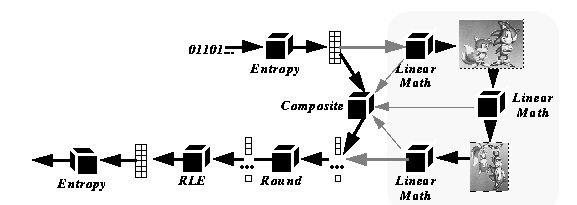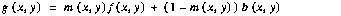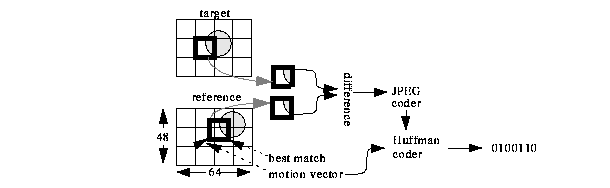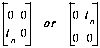Brian C. Smith is assistant professor of Computer Science at Cornell University. His research interests are multimedia applications and development tools, graphical user interfaces, computer graphics, and image processing. His previous research projects include the design and implementation of the CMT multimedia toolkit, a distributed programming extension to Tcl/Tk called Tcl-DP, and the Berkeley MPEG player.

A Survey of Compressed Domain Processing Techniques
Brian C. Smith

Processing video data is problematic due to the high data rates involved. Television quality video requires approximately 100 GBytes for each hour, or about 27 MBytes for each second. Such data sizes and rates severely stress storage systems and networks and make even the most trivial real-time processing impossible without special purpose hardware. Consequently, most video data is stored in a compressed format.

While compression reduces the storage and network costs, it increases the cost of processing since the data must be decompressed before it can be processed. The overhead of decompression is enormous: today's sophisticated compression algorithms, such as JPEG or MPEG, require between 150 and 300 instructions per pixel for decompression. This corresponds to a rate of 2.7 billion instructions for each second of NTSC quality video processed. Furthermore, the data must often be compressed after processing, which adds one to fifty times more overhead.

One way to circumvent these problems is to process the video data in compressed form. This technique reduces the amount of data that must be processed and avoids complex compression and decompression. Decreasing data volume has the side effect of increasing data locality and thus more efficiently uses processor cache, which further improves performance.

Over the past 4 years, many researchers have applied this idea to MPEG compressed audio, MPEG video, and motion JPEG video. This paper surveys these techniques.

JPEG is a standard for still image compression  that can achieve compression ratios as high as 50 to 1. When JPEG is applied to each frame in a video sequence, the result is called "motion JPEG" video compression. The baseline JPEG algorithm is outlined in figure 1. Briefly, JPEG converts an RGB image into the YUV color space, subsampling the chrominance (U and V) components. Each component is divided into 8x8 pixel blocks, which are transformed using a discrete cosine transform (DCT). The post-DCT 8x8 block is encoded as a one dimensional, 64 element vector using zig-zag scanning, a heuristic that places the low frequency coefficients, which are located in the upper left corner of the block, early in the vector. The vector is scaled by dividing each coefficient by a constant. The constant varies from element to element, and is proportional to the human eye's sensitivity to an error in each coefficient. The scaled vector has the following property: an error of up to one half can be introduced in each element without a noticeable difference in the reconstructed image. Consequently, the elements of the scaled vector are rounded to the nearest integer, a process called quantization. Experiments reveal that typically 55 to 60 of the 64 quantized elements are zero, so they are encoded using run-length encoding (RLE), and the result is entropy coded using either Huffman or arithmetic coding.Figure 1: JPEG Image Compression

In summary, JPEG is a seven step process:

1. The color space of the image is converted (RGB to YUV)
2. The discrete cosine transform (DCT) is applied to each 8x8 block of pixels
3. The result is transform into a 64 element vector using zig-zag coding
4. The vector is scaled.
5. The scaled vector is quantized (rounded to the nearest integer).
6. The quantized vector is run-length encoded (RLE)
7. The RLE vector is entropy coded.

Decompression is the reverse of compression. Two important facts about JPEG compression will be needed for the discussion in the next section. First, steps 1-4 are linear operators. Second, the RLE vector is a sparse-matrix representation of the scaled, quantized DCT vector.Figure 2: Spatial Domain Processing

We now turn to the problem of processing JPEG data. A naive approach would decompress the data, process it, and compress the result, as shown in figure 2. This strategy is called spatial domain processing. The basic idea of compressed domain processing is to convert the spatial domain process into its frequency domain equivalent. This conversion is accomplished by representing the spatial domain process and the transforms used in compression a linear operators, and form the composite operator. Compressed data is then processed by entropy decoding the bitstream to recover the sparse vector data, applying the compressed domain operator, and quantizing and compressing the result. Figure 3 shows this strategy.Figure 3: Compressed Domain Processing

Perhaps the simplest type of video processing that has been mapped into the compressed domain (for JPEG) is pixel arithmetic . This type of processing consists of four operations: scalar addition (add a constant to each pixel in an image), scalar multiplication (multiply each pixel in an image by a constant), pixel-wise addition (add the pixels in two images), and pixel-wise multiplication (multiply the pixels in two images). These operations can be implemented directly on the run-length coded, quantized vectors (the RLE vectors), which can be obtained by Huffman decoding the JPEG bitstream. It can be shown that scalar addition is equivalent to altering the DC component of each block (the first element in the RLE vector), scalar multiplication is equivalent to multiplying each element of the RLE vector by a constant, and pixel-wise addition is equivalent to adding two RLE vectors. Pixel-wise multiplication is more complex: it involves the multiplication all possible pairs in the two RLE vectors. Since the vectors are relatively sparse, this operation is not too expensive in the average case.

Pixel arithmetic can be used to implement a number of operations. For example, a cross-fade between two video sequences is described by the function(EQ 1)

where g is an image in the output sequence, f1 and f2 are images in the source sequences, and α is a parameter that varies from 0 (beginning of the cross-fade) to 1 (end of the cross-fade). The implementation of this operation on compressed domain data is straightforward, since it involves only scalar multiplication and pixel-wise addition, and the compressed domain operator is about 100 times faster than its spatial domain equivalent .

Another application of pixel arithmetic is image composition. A familiar example of this operation is overlaying a forecaster on a weather map. The overlay is accomplished using three images: a background image (the weather map), a foreground image (the forecaster), and a masking image, which is a bi-level image that has a pixel value of 1 if the corresponding pixel in the output should be taken from the foreground image, 0 if it should be taken from the background. The output image is computed using the following function:(EQ 2)

where g is the output image, m is the mask, f is the foreground, and b is the background. Since the only operations that are used in this processing are pixel-wise multiplication, scalar addition, and pixel-wise addition, the operation can be mapped into the compressed domain. For typically applications, the compressed domain operator is about 10 to 50 times faster than the corresponding spatial domain implementation .

Two problems arise with image composition in the compressed domain. First, there is no known simple way to generate the mask image (called the matte) in the compressed domain. In television studios, these images are generated in the spatial domain using chroma-keying: the forecaster is placed in front of a bright green or blue screen, and that color is used to generate the mask image. No one has determined how to generate a matte directly on compressed data.

A second problem is that the edges of the foreground object and the edges in the matte are often highly correlated, since they both lie on the foreground object's boundary. The presence of edges increases the size of the RLE vectors, which makes the masking operation can be relatively expensive for the foreground image, since the cost of implementing a pixel-wise multiply on two RLE vectors is proportional to the product of the size of the two vectors. Often, the compressed domain operation is slower for these blocks than the spatial domain equivalent. But since there are relatively few edge blocks in typical mattes (maybe 10%), operating in the compressed domain is many times faster than in the spatial domain.

The four operations described above, pixel and scalar addition and multiplication, are local operations: the value of a pixel in the output is uniquely determined by the value of the corresponding pixel(s) in the source image(s). A more complicated type of processing uses global operations, where the value of a pixel in the output image is a function of many pixels in the source image. Linear global operations, where each output pixel is a linear combination of input pixels, can be implemented in the JPEG domain .

The basic idea of compressed domain global processing is to factor JPEG into steps that can be written mathematically as linear operators (scaling, DCT, zigzag scanning) and steps that can not (quantization, run-length and Huffman coding). Once JPEG compression, JPEG decompression, and the spatial domain operation (i.e., the processing) are written as linear operators, they can be combined. Two complications muddy this otherwise simple idea. First, the resulting operators can be more expensive to implement than the spatial domain equivalent, both in memory and operation count. This defect can be corrected using a scheme called condensation to approximate the compressed domain operator by a sparse operator. The image that results from using the sparse operator is nearly indistinguishable from the correct result, but its computation is much faster: about twice the time required for Huffman compression and decompression .

The second complication is that mapping global operations into the compressed domain is complicated because the spatial domain operators may cross block boundaries. For example, a common way to implement the operation of smoothing an image is to replace each pixel by an average of its immediate neighbors. Since an 8x8 pixel block in the output image is a function of a 10x10 block of pixels in the source image, the coefficients of the corresponding output RLE vector will be a complex linear combination of coefficients from nine RLE vectors in the input. Computing the appropriate linear combination is tractable if the spatial domain operators, and the operators corresponding to the compression and decompression, are written as high dimensional matrices. This technique gives rise to fairly complicated index gymnastics, but the calculation is straightforward.

Global operations in the compressed domain are several times faster than their spatial domain equivalents, but this efficiency comes at the cost of increased complexity of implementation. Moreover, this method of mapping global operations into the compressed domain results in impractically large data structures (tens of megabytes) unless the operation exhibits special symmetry.

These limitations have prompted other researchers to examine special cases that can be implemented efficiently. Two examples are the inner block operations discussed by Shen and Sethi , and the scaling operations derived by Natarajan and Bhaskaran .

Natarajan and Bhaskaran showed that the operation of shrinking an image by a factor of two could be implemented in the compressed domain using only shifts and adds. His work maps the scaling operator directly into the compressed domain and then factors the result into a sequence of operations with many common sub-expressions that consist of multiplies and divides by powers of two. The speed of the resulting operations is approximately 5:1, and the image quality is very high.

Sethi and Shen examined the special case of inner block transforms (IBTs), which are a form of factoring global operations. In IBTs, the coefficients in an output block is a function a single block in the source, but the combination of pixels in a block is a global operation. When IBTs are combined with pixel addition, IBTs can be used to implement a wide variety of operations, including image rotation.

Manipulating pixel values in the compressed domain provides the basis for implementing special effects, but does little to address image and video content. For example, it would be advantageous to search an image/video database using only compressed domain operations. Several researchers have pursued this idea. Two notable contributions are Seales work on object recognition  and Arman's work on cut detection .

Seales examined searching a database of "mug shots" in the compressed domain using an eigenspace method. The basic idea of this work is to represent a large-dimensional object (an image) in a lower-dimensional subspace. The basis vectors for the subspace are images, called "eigen-faces", capture the most important features of the images in the database. Once the eigen-faces are computed, a linear combination of the eigen-faces is computed for each image in the database that closely approximates the image. The coefficients of this linear combination are used as an index in the database. To locate an image in the database, the eigen-face coefficients of the target image (the one you are searching for) are computed and compared against the database index. The matches are sorted and returned to the user.

Seales's method computes the eigen-faces directly on the RLE vectors, rather than pixel values. This allows the target image's index to be computed without decompressing the image, a significant savings. Seales showed that image searching using eigen-faces in the compressed domain was just as effective as in the image domain for moderate quality images, proving the validity of the technique and showing that the information lost during compression was insignificant.

Arman, Hsu, and Chiu examined the problem of cut detection in the compressed domain. The problem of cut detection is this: given a video, segment it into a sequence of scenes or "shots." The shot boundaries are called cuts, and are the results of editing the raw video footage. Arman showed how to perform cut detection directly on JPEG compressed video data. His approach compares a subset of coefficients selected from RLE blocks which are extracted from frames of a video sequence. The position of the extracted RLE blocks, as well as the subset of coefficients chosen, remains constant throughout the sequence. The set of coefficients thus obtained is used to form a normalized vector that represents the frame. Two frames are compared by computing the dot product of their normalized representative vectors. When the dot product is sufficiently small, a scene cut is declared. The procedure is found to be fast, simple, and effective.

Although JPEG is very effective at compressing still images, further compression can be achieved using the MPEG video standard . MPEG augments JPEG with motion compensation, a form of differential encoding, and achieves compression ratios as high as 200 to 1. In MPEG, each frame in a video sequence is encoded as either an I-frame, a P-frame, or a B-frames. A typical frame sequence is shown in figure 4, along with the inter-frame dependencies. I-frames are independent of other frames, and their encoding is almost identical to JPEG.Figure 4: MPEG Dependencies

B-frames and P-frames are encoded as a difference from nearby I- or P-frames (called reference frames). P-frame encoding is shown in figure 5. MPEG partitions a P-frame into 16x16 pixel macroblocks. Each macroblock in the P-frame, called the target macroblock, is encoded by searching for a macroblock in the reference frame (the most recent I- or P-frame), typically using the mean squared error or mean absolute error as a metric. The difference in coordinates between the target macroblock and its best match is called the motion vector, and their pixel-wise difference is called the error term. The error term is compressed using JPEG and stored along with the motion vector in the MPEG bitstream. B-frame compression is nearly identical to P-frame compression, except that two reference frames (one from the earlier in the sequence and one from later) can be used. This extra degree of freedom can result in very high compression ratios, as high as 500 to 1.Figure 5: P-frame encoding

To decode an MPEG frame, all relevant reference frames must first be decoded. Then each error term is decompression, and the result is added pixel-wise to the 16x16 pixel block in the reference frame specified by the motion vector.

The processing in the previous section used JPEG compressed image and video data. A natural question is "can these techniques be extended to MPEG video data?" Since MPEG uses a more complex compression strategy than JPEG, the extension is non-trivial. Recall that MPEG defines three types of frames, I-, P-, and B-frames. I-frames are very similar to JPEG frames, while P- and B-frames use motion compensation. This motion compensation poses the most difficult problem for operating on MPEG data, since the motion vector may refer to a block of pixels that is not aligned on a macroblock boundary.Figure 6: Reference Macroblock (R), motion vector, and original macroblocks

Chang's solution to this problem is to convert P- and B- frames into I-frames, then process the resulting frames using strategies similar to those of JPEG frames [4, 5]. Converting a P-or B-frame into an I-frame requires computing DCT coefficients of a motion compensated macroblock from the motion vector and the coefficients in the reference frame. Chang showed how to compute these coefficients by pre-multiplying and post-multiplying the reference macroblocks with appropriate matrices. Suppose in figure 6, R is the macroblock in the reference frame that we wish to derive from the four original macroblocks P1... P4. In the spatial domain, this conversion is expressed by the following equation:(EQ 3)

where Sij are matrices likeEach In is an identity matrix of size n.

Applying the DCT to each side of this equation, we havewhere DCT(R) denotes the DCT on matrix R. Once the DCT coefficients of R have been calculated, the result can be scaled and added to the error term in the compressed domain using pixel addition.

Once the problem of P- or B- to I-frame conversion has been solved, it becomes possible to transcode MPEG data to JPEG data in the compressed domain. The advantage of this technique would be that existing JPEG hardware could be used to display MPEG data. Smith and Arachya have studied this problem recently , with promising results: MPEG to JPEG conversion can be performed at rates four to five times faster in the compressed domain than in the spatial domain. Their method caches converted motion-compensated macroblocks for additional performance.

Yeo and Liu have studied the problem of cut detection on MPEG data. Their approach analyzes the difference image between successive frames in the spatial domain using DC-images, which are obtained by shrinking an image by a factor of 8 in each dimension. The DC-image is obtained from I-frames by taking the first coefficient in an RLE block. They use a clever approximation of Chang's technique to compute the DC-images, for P- and B-frames. The cost to compute each pixel in the DC-image using their technique is at most 4 multiplications.

The MPEG audio encoder achieves compression ratios between 6 and 12 to 1 . MPEG audio converts a group samples into 32 equal width frequency bands using the subband analysis. For example, CD quality audio digitized at 44.1 kHz would be divided into 32 bands, each 689 Hz wide. The result of the analysis is 32 amplitude coefficients, one for each band, that indicates the strength of that band. Each coefficient is then quantized using masking.

Masking is a psychoacoustic effect where larger amplitude signals obscures lower amplitude signals. Studies have shown that masking decreases with distance in the frequency domain. This fact means that, in MPEG audio, an amplitude coefficient with a large value will tend to render a neighboring coefficient with a small value inaudible. MPEG uses this fact to represent the smaller, neighboring coefficient with fewer bits than its large neighbor. The exact number of bits assigned to each coefficient is determined by a psychoacoustic model. The model also computes a scaling factor for each band, which is essentially an exponent which is used to expand the quantized sample to the necessary range. The quantized sample, bit allocations, and scaling factors comprise the resulting bitstream. The MPEG audio compression process is illustrated in figure 7.Figure 7: MPEG Audio Encoding

Few researchers have explored the possibility of directly manipulating MPEG compressed audio data. The area has attracted little attention because many researchers believe that less significant gains are possible because audio data rates are lower; CD-quality audio corresponds to a data rate of 192 KBytes/sec. But the sophisticated compression and decompression techniques used in MPEG audio compression make real-time processing of compressed audio data impossible on current generation machines.

To date, the only work known by the author is that of Broadhead and Owen, who studied the problem of gain control, mixing, and equalization on MPEG audio streams . A detailed discussion is beyond the scope of this survey, but they report that mixing in the compressed domain is five times faster than the equivalent uncompressed domain operation, and that the resulting bitstreams are very high quality.

This paper has surveyed techniques for processing audio, image, and video data in the compressed domain. The advantage of compressed domain operations is that they can often be performed many times faster than their spatial domain counterparts, and that they process less data, which increases locality and lowers bandwidth requirements.

Several open problems remain. There are no known techniques for performing non-linear operations in the compressed domain. For example, chroma-keying in the compressed domain is impossible, as is any sort of color map modification such as gamma correction. Another problem is that the techniques that process MPEG produce JPEG data (or MPEG with all I-frames) as a result. The techniques would be significantly more attractive if motion-compensated MPEG data, with B- and P-frames, could be calculated directly in the compressed domain.

The motion compensation data present in MPEG video could be used in other ways. For example, many vision algorithms use motion information in their processing. Since this information is effectively pre-computed in MPEG data, such algorithms might work particularly well in the compressed domain. Finally, direct manipulation of compressed audio data deserves more attention.

 Soam Acharya, personal communication

 Farshid Arman, Arding Hsu, Ming-Yee Chiu, Image Processing on Compressed Data for Large Video Databases, Proceedings of the First ACM International Conference on Multimedia, August 1993, Anaheim, Calif.

 M. Alexander Broadhead, Charles B. Owen, Direct Manipulation of MPEG Compressed Digital Audio, to appear in Proc. of the Third ACM International Conference on Multimedia, San Francisco, CA, November 5-9, 1995

 Shih-Fu Chang, Wen-Lung Chen and David G. Messerschmitt, Video Compositing in the DCT Domain, IEEE Workshop on Visual Signal Processing and Communications, Raleigh, NC, pp. 138-143, Sep. 1992.

 Shih-Fu Chang and David G. Messerschmitt, A New Approach to Decoding and Compositing Motion Compensated DCT-Based Images, IEEE Intern. Conf. on Accoustics, Speech, and Signal Processing, Minneapolis, Minnesota, pp. V421-V424, April, 1993.

 A.J.M. Houtsma, Psychophysics and Modern Digital Audio Technology, Philips Journal Research, Vol 47, 1992, pp 3-14.

 K. Fukunaga, Introduction to Statistical Recognition, Academic Press, 1990.

 D. Le Gall, MPEG:A Video Compression Standard for Multimedia Applications, Communications of the ACM, April 1991, vol.34, (no.4):46-58

 B. Natarajan, V. Bhaskaran, A Fast Approximate Algorithm for Scaling Down Digital Images in the DCT Domain, to appear in IEEE International Conference on Image Processing, Oct. 23-26, 1995, Washington D.C.

 William B Pennebaker, JPEG still image data compression standard, Van Nostrand Reinhold, New York, 1992.

 W. Brent Seales, Matthew D. Cutts, Vision and Multimedia: Object Recognition in the Compressed Domain, University of Kentucky Technical Report, Oct 1995, seales@dcs.uky.edu

 Bo Shen and Ishwar K. Sethi, Inner-block operations on compressed images, to appear in Proc. of the Third ACM International Conference on Multimedia, San Francisco, CA, November 5-9, 1995

 Brian C. Smith, Lawrence A. Rowe, Algorithms for Manipulating Compressed Images, IEEE Computer Graphics and Applications, Sept. 1993, vol.13, (no.5):34-42.

 Brian C. Smith, Fast Software Processing of Motion JPEG Video, Proc. of the Second ACM International Conference on Multimedia, San Francisco, CA, October 15-20, 1994.